Functions: Using Patterns in Delayed Assignment (:=)

Defining Functions with Patterns (This is an important section to understand well)

Defining functions with patterns probably combines the most useful aspects of Mathematica.  Define a function that takes patten matching x as its first argument and an argument matching n as its second argument and returns x to the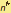power: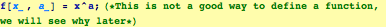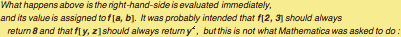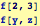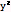This works fine, but suppose we had defined  x ahead of time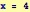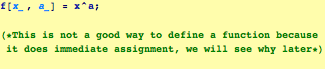RHS evaluated, it is x^(second argument) which is currently 4^(second argument)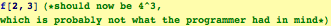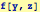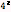Delayed Assignmet (:=)

The problem with the above functions is that the evaluation is made immediately.  For a function, the argument serves as a placeholder for a future calculation.  You do not wish to have the right–hand–side evaluated until the actual values of left–hand–side are specified.  The remedy is to use delayed assignment :=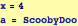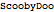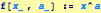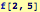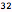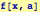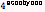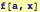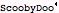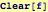This is the paradigm for defining functions: A Pattern with a Delayed Assignment with that Pattern:
myFunction[pattern_] := pattern^2